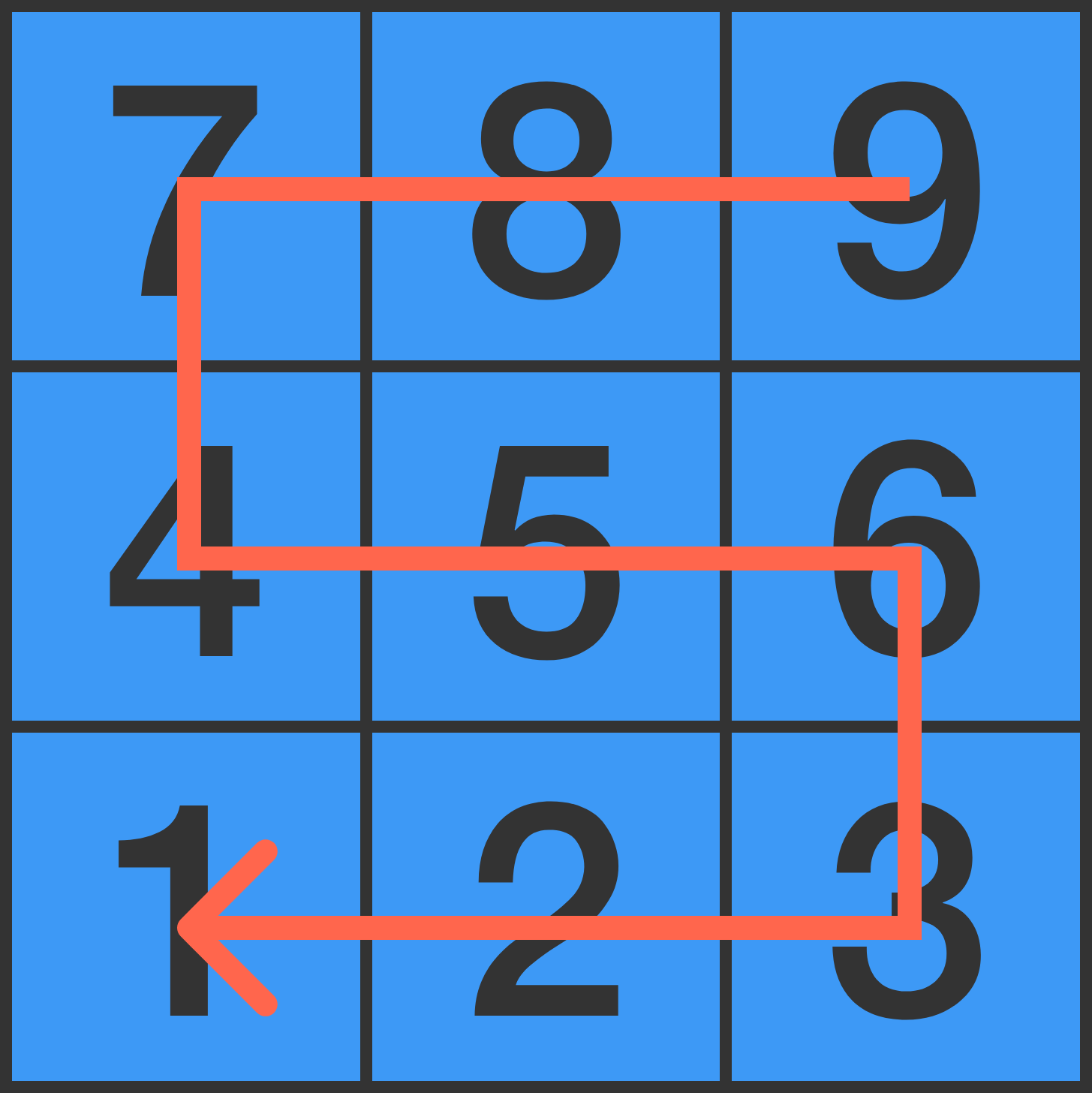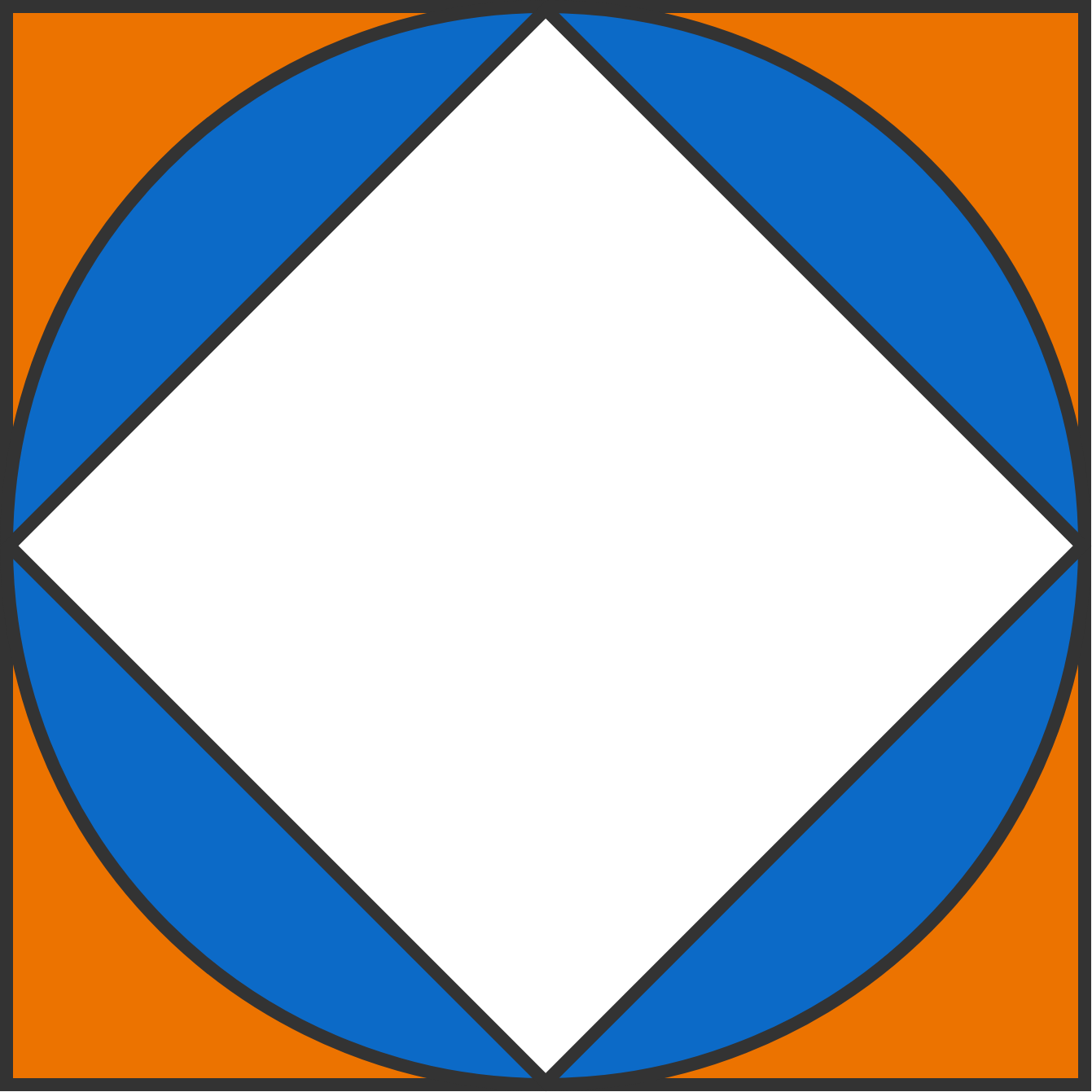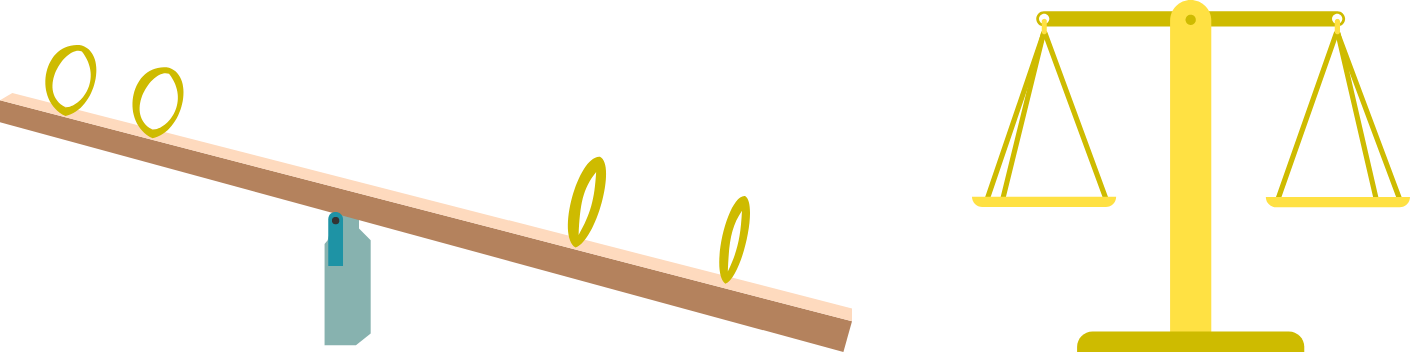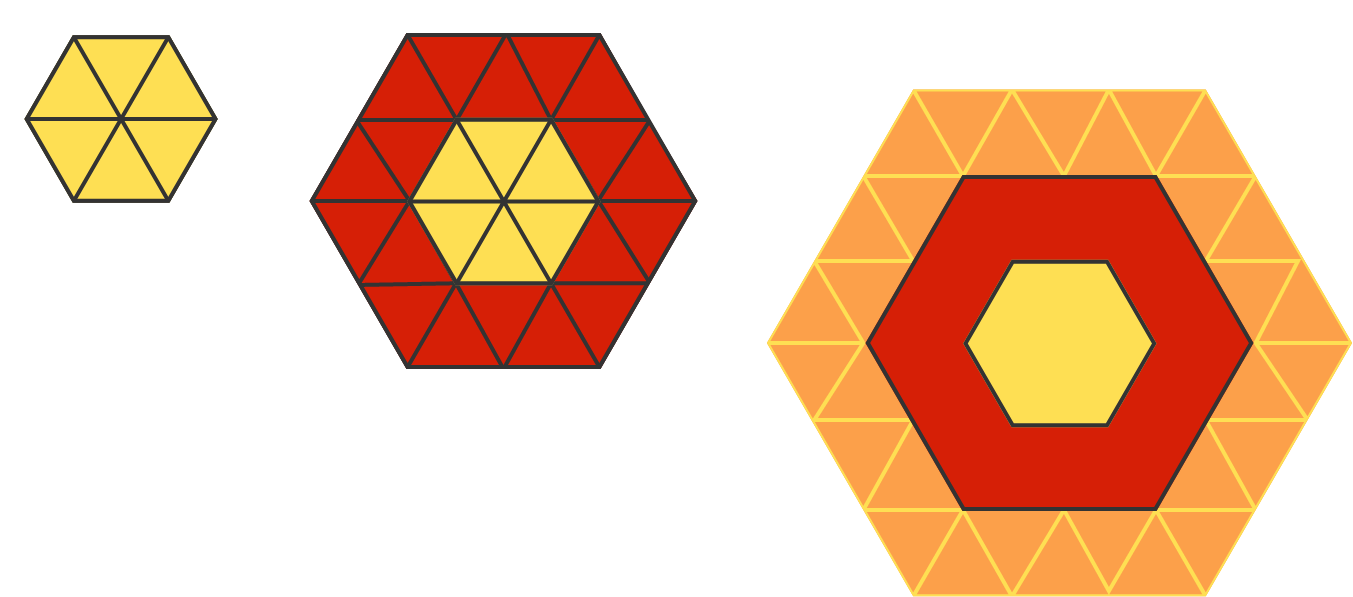# Problems of the Week

Contribute a problem

# 2018-05-28 Basic

On this number pad, a nine-digit number is made by walking through each digit once, starting from a random digit and only moving to adjacent digits.That number is then divided by its first digit. In this example, ${ \color{#EC7300}9}87456321 ÷ {\color{#EC7300}9} = 109717369.$ Will this method always result in an integer?

True or False?

If $(a-b) \times (a-b) = (c-b) \times (c-b),$ then it must be true that $a=c.$

Bonus: If you think it's true, prove it; if you think it's false, find a counterexample.A white square is inscribed in a blue circle, which is inscribed in a larger orange square.

Which area is larger, orange or blue?

Why is a seesaw always tilted to one side, while an empty beam balance is always balanced horizontally?

A. The center of mass of a seesaw is slightly above the hinge point, while that of a beam balance is slightly below the hinge point.

B. The center of mass of a seesaw is slightly below the hinge point, while that of a beam balance is slightly above the hinge point.The diagram shows how identical equilateral triangles can be tiled to make a regular hexagon with no leftover tiles.

Which of the following numbers of triangles can also do this?Regular hexagons can be made with 6 tiles, 24 tiles, and 54 tiles.

×